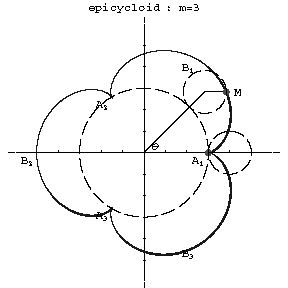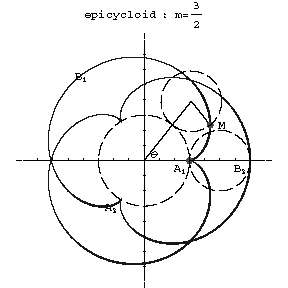# Epicycloid

A planar curve given by the trajectory of a point on a circle rolling on the exterior side of another circle. The parametric equations are:

$$x=(r+R)\cos\theta-r\cos\left[(r+R)\frac\theta r\right],$$

$$y=(r+R)\sin\theta-r\sin\left[(r+R)\frac\theta r\right],$$

where $r$ is the radius of the rolling and $R$ that of the fixed circle, and $\theta$ is the angle between the radius vector of the point of contact of the circles (see Fig. a, Fig. b) and the $x$-axis.Figure: e035860a

$m=3$Figure: e035860b

$m=3/2$

Depending on the value of the modulus $m=R/r$, the resulting epicycloid has different forms. For $m=1$ it is a cardioid, and if $m$ is an integer, the curve consists of $m$ distinct branches. The cusps $A_1,\ldots,A_m$ have the polar coordinates $\rho=R$, $\phi=\pi2k/m$, $k=0,\ldots,m-1$. The vertices $B_1,\ldots,B_m$ of the curve have the coordinates $\rho=R+2r$, $\phi=\pi(2k+1)/m$. When $m$ is a rational fraction, the branches intersect each other in the interior; when $m$ is irrational there are infinitely many branches and the curve does not return to a point $M$ describing a position obtained previously; for rational $m$ the epicycloid is a closed algebraic curve. The arc length from the point $A_1$ is:

$$s=8Rm(1+m)\sin^2\frac\theta4,$$

and from $B_1$ it is

$$s=4Rm(1+m)\cos\frac\theta2.$$

The area of a sector bounded by two radius vectors of the curve and its arc is

$$S=m\pi(R+mR)(R+2mR).$$

$$r_k=\frac{4Rm(1+m)}{2m+1}\sin\frac\theta2.$$